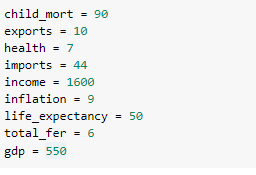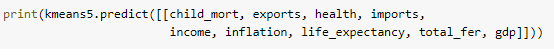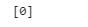• No products in the cart.

# K-means Clustering in Python

## Introduction

Clustering or cluster analysis is an unsupervised learning problem. It deals with finding groups of objects such that the objects in a group are similar (or related) to one another and different from (or unrelated to) the objects in other groups. It is often used as a data analysis technique for discovering interesting patterns in data, such as groups of customers based on their behavior.

So, in this article, we’ll be taking a look at the step-by-step implementation of the K-means clustering algorithm. However, before we dive into the coding part, I’ll provide a basic overview of how the algorithm works, so you don’t get lost in the implementation.

By the end of the article, you’ll have all your concepts clear about what K-means clustering is and how it helps us solve major problems easily. So, make sure you give the tutorial a thorough read. Let’s start!

## Types of Clustering

There are mainly three types of clustering techniques which are explained below:

• Partitional ClusteringnA division of data objects into non-overlapping subsets (clusters) such that each data object is in exactly one subset.
• Hierarchical ClusteringnA set of nested clusters organized as a hierarchical tree. They may correspond to meaningful taxonomies.
• Density-based ClusteringnIn this method, the clusters are created based upon the density of the data points which are represented in the data space. The regions that become dense due to the huge number of data points residing in that region are considered clusters.

## Using the Model to Cluster New Data Points

Now that we have accomplished the task of training our K-Means clustering model, let’s see how we can easily use it in real-life to cluster any new set of data. Our aim here is to input any random set of values for all the features available and let the model decide which cluster does our point belongs to.

So, how do we do it? Well, we simply feed our model the values we want to cluster, and it will predict the cluster those values belong to. This can be done using sklearn’s predict function. However, let’s make some variables to hold the feature values first so they don’t get confusing.?

Now that the values make sense, let’s predict the cluster now.?

Let’s run this cell and see what we get.?

Great! the values we used belong to cluster number 0. This way, we can use any set of values we want and use our model to assign them a suitable cluster.

## Conclusion

Even though K-means algorithm is highly easy to implement and comprehend, it doesn’t always give a perfect output. The disadvantage of K-means lies in the fact that you manually have to select a value of K. It is also dependent upon the initial cluster centroid values. K-means has trouble clustering data where clusters are of varying sizes and densities. Outliers in your data can also greatly impact your clusters.

So, unless you have such problems available in your dataset, it’s a great unsupervised learning algorithm that you can use to develop a clustering model.

I hope you had all your concepts cleared about how K Means Clustering can be implemented using scikit-learn. If you want to get your hands on the full code used in this tutorial, don’t hesitate to get it from my GitHub here.

August 7, 2021